# Common fractions and decimals#### Everything You Need in One Place

Homework problems? Exam preparation? Trying to grasp a concept or just brushing up the basics? Our extensive help & practice library have got you covered.#### Learn and Practise With Ease

Our proven video lessons ease you through problems quickly, and you get tonnes of friendly practise on questions that trip students up on tests and finals.#### Instant and Unlimited Help

Our personalized learning platform enables you to instantly find the exact walkthrough to your specific type of question. Activate unlimited help now!

0/5
##### Intros
###### Lessons
1. Introduction to Common Fractions and Decimals:
2. How are decimals related to fractions?
3. How to convert decimal tenths into decimal fractions ($\large \frac{1}{10}$)
4. How to convert decimal hundredths into decimal fractions ($\large \frac{1}{100}$)
5. How to convert between decimals and common fraction halves ($\large \frac{1}{2}$)
6. How to convert between decimals and common fraction quarters ($\large \frac{1}{4}$)
0/13
##### Examples
###### Lessons
1. Writing common fractions as decimals
Write the following fractions as decimals
1. Unit fractions:
2. Decimal fractions:
3. Common fractions related to $\large \frac{1}{2}$ or $\large \frac{1}{4}$:
2. Fraction and figure equivalents for decimals
Complete the table for the equivalent decimal, fraction, and shaded in figure:
1.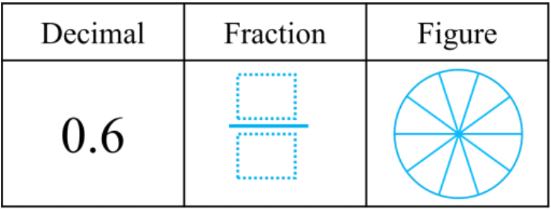2.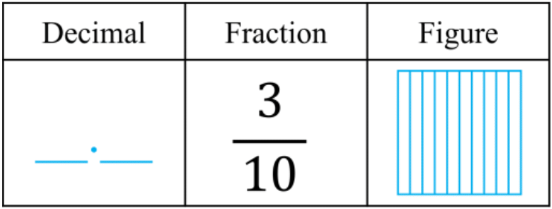3.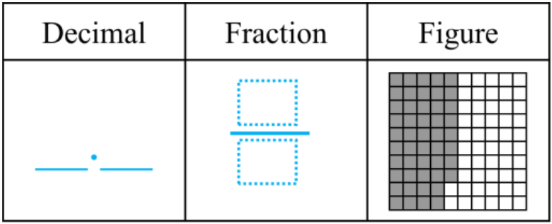4.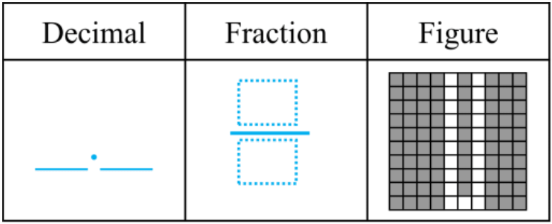3. Fraction and figure equivalents for decimals
Complete the table for the equivalent decimal, fraction, and shaded in figure:
1. 0.28 = $\large \frac{}{100}$ = $\large \frac{20}{100}$ + $\large \frac{}{100}$ = $\large \frac{}{10}$ + $\large \frac{}{100}$
2. 0.51 = $\large \frac{}{100}$ = $\large \frac{50}{100}$ + $\large \frac{}{100}$ = $\large \frac{}{10}$ + $\large \frac{}{100}$
3. 0.73 = $\large \frac{?}{?}$ = $\large \frac{}{100}$ + $\large \frac{}{100}$ = $\large \frac{}{10}$ + $\large \frac{}{100}$
4. 0.49 = $\large \frac{?}{?}$ = $\large \frac{?}{?}$ + $\large \frac{?}{?}$ = $\large \frac{?}{?}$ + $\large \frac{?}{?}$
4. Common fractions and decimal number lines
Using common fractions and decimals:

A:$\large \frac{25}{100} \qquad \;$ B:$\large \frac{7}{10} \qquad \;$ C:$\large \frac{90}{100} \qquad \;$ D:$\large \frac{5}{10} \qquad \;$ A:$\large \frac{75}{100} \qquad$
1. Draw each fraction as a point on the number line. Label each point with its corresponding letter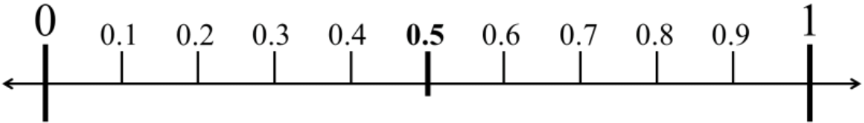2. Which fractions can be rewritten in lowest forms as common fraction halves and quarters?
0%
##### Practice
###### Topic Notes

In this lesson, we will learn:

• How to convert between decimals and decimal fractions (tenths and hundredths)
• How to convert between decimals and unit fractions
• How to convert between decimals and fraction halves and quarters

Notes:

• Decimals can be converted into fractions and vice versa
• Recall that place values are related to their neighbors by a factor of 10

•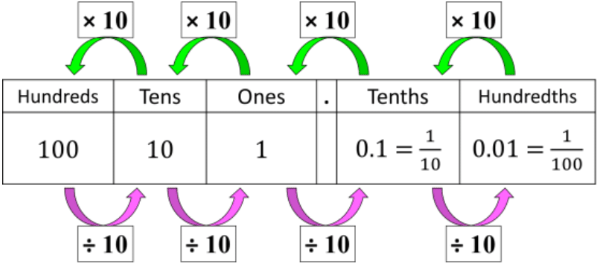• Decimals can be easily converted into decimal fractions
• Decimal fractions have denominators that are powers of 10
• Decimal tenths are fractions out of 10
• Ex. 0.3 = $\large \frac{3}{10}$; 3 tenths = 3 out of 10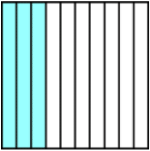• Decimal hundredths are fractions out of 100
• Ex. 0.22 = $\large \frac{22}{100}$; 22 hundredths = 22 out of 100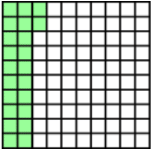• Decimal hundredths can also be expressed as a sum of fractions, because of equivalent decimals (i.e. 0.2 = 0.20)
• 0.22 = $\large \frac{22}{100}$ = $\large \frac{20}{100}$ + $\large \frac{2}{100}$ = $\large \frac{2}{10}$ + $\large \frac{2}{100}$

• Two type of common fractions have a denominator of 2 or 4

 Type Fraction Decimal Value "half" $\frac{1}{2}$ = $\frac{50}{100}$ = $\frac{5}{10}$ 0.5 "quarter" $\frac{1}{4}$ = $\frac{25}{100}$ 0.25